Time series analysis is a statistical technique to analyze the pattern of data points taken over time to forecast the future. The major components or pattern that are analyzed through time series are:

• Trend Increase or decrease in the series of data over longer a period.
• Seasonality Fluctuations in the pattern due to seasonal determinants over a short period.
• Cyclicity Variations occurring at irregular intervals due to certain circumstances.
• Irregularity Instability due to random factors that do not repeat in the pattern.

# Time Series Analysis for Data-driven Decision-Making

Time series analysis helps in analyzing the past, which comes in handy to forecast the future. The method is extensively employed in a financial and business forecast based on the historical pattern of data points collected over time and comparing it with the current trends. This is the biggest advantage used by organizations for decision making and policy planning by several organizations.

# Applicability of Time Series Analysis

Time Series analysis is “an ordered sequence of values of a variable at equally spaced time intervals.” It is used to understand the determining factors and structure behind the observed data, choose a model to forecast, thereby leading to better decision making.

The Time Series Analysis is applied for various purposes, such as:

• Stock Market Analysis
• Economic Forecasting
• Inventory studies
• Budgetary Analysis
• Census Analysis
• Yield Projection
• Sales Forecasting

and more.

# Time Series Analysis - Statistical Elaboration and Significance

Time series refers to a series of data indexed data in temporal order. Time series analysis is the technique of analyzing time-series data to pull out the statistics and characteristics related to the data.

There are two methods for the time series analysis:

• Frequency Domain Method It includes wavelet analysis and spectral analysis.
• Time Domain Method It includes cross-correlation and autocorrelation.

The time series analysis technique can be further divided into the following:

• Parametric Approach The process assumes that the underlying stationary process follows a structure that can be explained in small parameters.
• Non- parametric Approach It estimates the covariance instead of assuming any structure.

The time series analysis can also be classified into linear, non-linear, univariate, and multivariate.

The method utilizes historical data to analyze patterns and trends, issues related to seasonality and cyclical fluctuation to forecast the future. It is widely popular in investment to track the price of a security over a period.

It also determines the changes of data due to change in a variable over the same time. For instance, the change in stock share price depending on an economic variable like the unemployment rate can also be recorded through time series analysis. It brings out the pattern of a situation reflecting the relationship between the data point and the variable.

# Time Series Modeling

There are different models of time series analysis to bring out the desired results:

### ARIMA Model

ARIMA stands for Autoregressive Integrated Moving Average model, which is a type of regression analysis that measures the influence of one dependent variable corresponding to changing variables. The model is used to forecast moves in the financial market, analyzing the differences in values in a series rather than the actual values.

ARIMA can be classified into three components:

AR stands for Autoregression, where the dependent relationship is used between observation and many lagged observations.

I stands for Integrated, where raw observation is differenced and is used to make the time series stationary.

MA or the Moving Average, use the dependency between observation and residual error.

Each component is defined as a parameter which is substituted as integers to indicate the usage of the ARIMA model.

Following are the parameters:

p – denotes lag order or the number of lag observations.

d – denotes the degree of differencing or the number of times the raw observations are differenced.

q – denotes the order of moving average or the size of the moving average window.

### ARIMA and Stationarity

A stationary model is when there is consistency in data over a period. ARIMA model makes the data stationary by differencing. For example, most of the economic data reflects a trend. Differencing of data removes the trends to make it stationary.

Below is an example of monthly index values that are analyzed monthly. The plot suggests that the data is non-stationary, showing an upward trend. Therefore, the ARIMA model can analyze and forecast and make the data stationary:### ARIMA and Stationarity

The Autoregressive (AR) model forecasts the future, deriving the behavioral pattern from the past data. It is useful when there is a correlation between the data in a time series. The model is based on the linear regression of the data in the current time series against the previous data on the same series.

Below is an example of Google stock price from 2-7-2005 to 7-7-2005, which has n = 105 values. The data is analyzed to identify the AR model. In the figure below, the plot represents stock prices vs. time.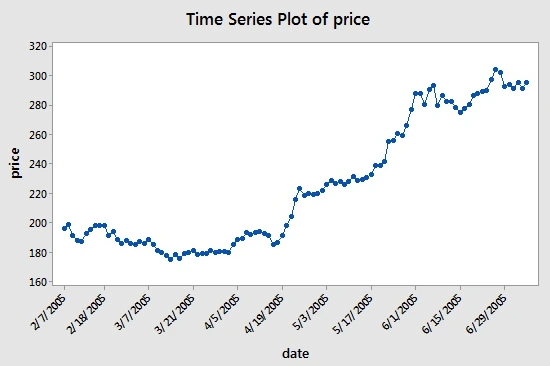https://newonlinecourses.science.psu.edu/stat501/node/358/

The values closely follow each other, suggesting the need for AR model. The next plot shows a partial autocorrelation for the data: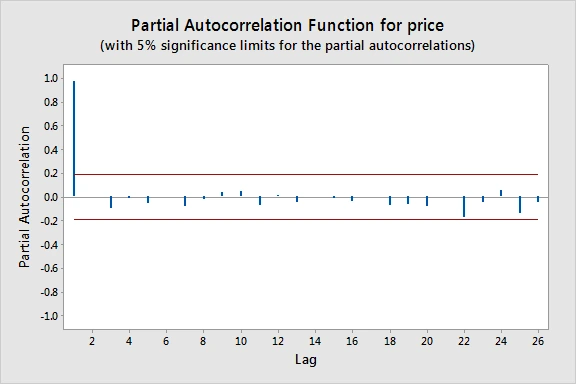https://newonlinecourses.science.psu.edu/stat501/node/358/

We can create a lag-1 price variable and compare the scatter plot with the lag-1 variable: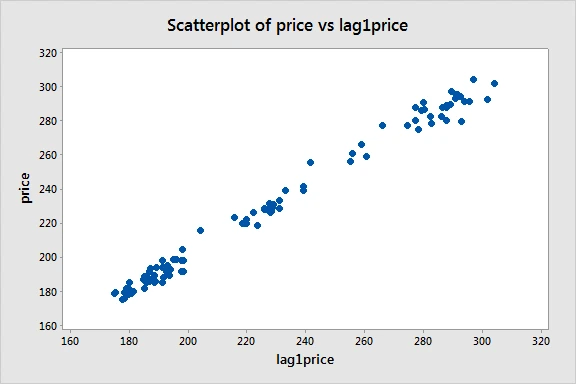https://newonlinecourses.science.psu.edu/stat501/node/358/

A moderate linear pattern can be observed, suggesting the suitability of the first-order AR model.

### Moving Average Model (MA)

Moving the average process is used to model the univariate time series. The model defines that the output variable is linearly contingent on present and the past data of a time series. It uses past errors in the forecast in a regression instead of the past value of the forecast variable.

Moving average helps in reducing the “noise” in the price. If, in a chart, the moving average in angled upward, it quite suggests a rise in price, if it points downward, it indicates the price is going down, and in case it is moving sideways, the price is likely to be in range.

In an upward trend of 50, 100, or 200 days, the moving average may support like a floor on which the price bounces. Check the chart below form GE.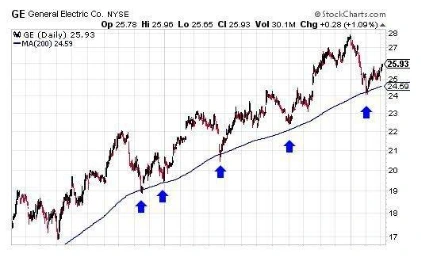https://pbs.twimg.com/media/D8j-XM-VsAEtrYp.jpg

# How to Choose the Model

Time-series analysis use techniques to derive insights on autocorrelated data, correlation of a series. However, the models need to be chosen properly to get accurate results. To choose the models, we must have clear objectives:

• What are we forecasting?
• What are the success parameters?
• What is then forecast horizon?

The next step is to analyze if the dataset is stationary or having a constant variable over time or non-stationary. This will help in determining the suitable forecasting model.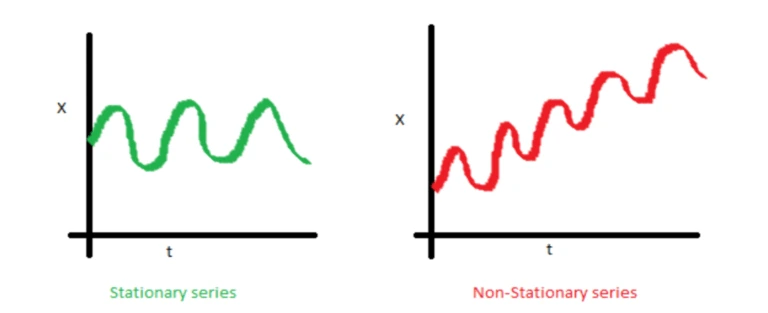https://www.datalytyx.com/choosing-the-right-forecast-model-for-time-series-data/

# Time Series in Relation to Python and R

R is a programming language used in statistical computing. The R software environment is a larger ecosystem and is functional with in-built data analysis methods.

Python, on the other hand, is language with statistical modules for general purpose. It is more object-oriented and has “main” packages for data analysis.

Though there are clear points of differences, both R and Python continue to be stronger and popular and have common syntax for several tasks.

Below is an example of time series analysis using R:

Extracting the trend, seasonality and error in European Stock Market -

The decompose() and forecast::stl() splits the time series into seasonality, trend and error components.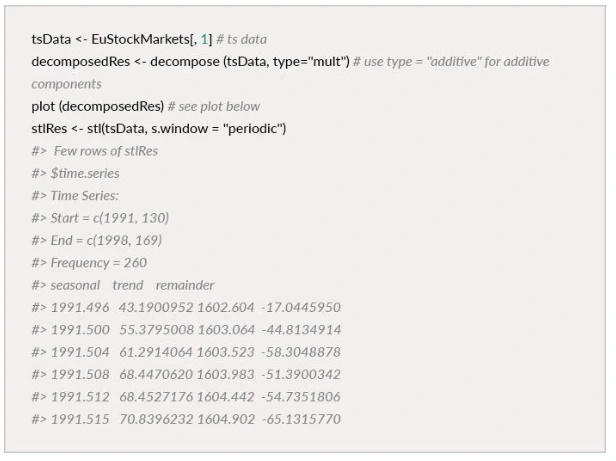The chart below shows a significant autocorrelation of lags on x-axis for AirPassengers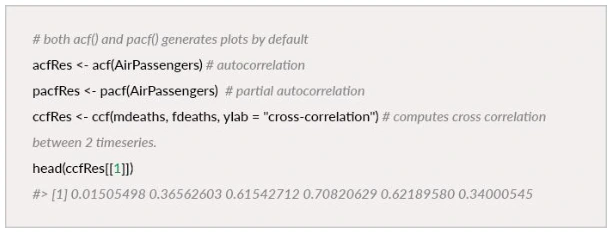### Testing if the time series is stationary

Augmented Dickey-Fuller Test (ADF test) is used where a less than 0.05 p-value indicates that the time series is stationary.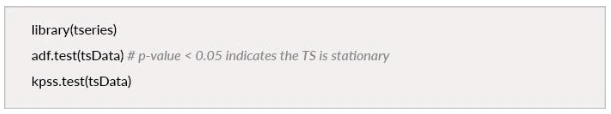Below is an example of a time series analysis of furniture sales using Python:Data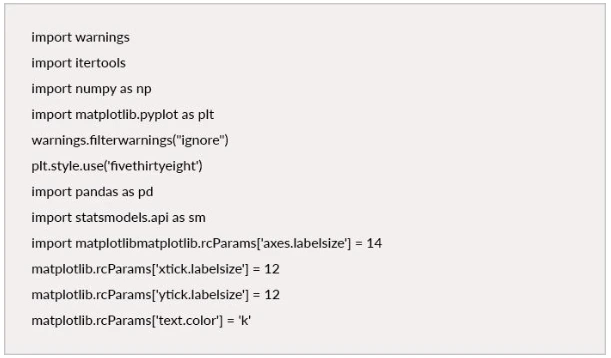Time series analysis and forecasting of furniture sales were done:Furniture sales data for 4-years: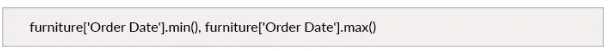### Timestamp(‘2014–01–06 00:00:00’), Timestamp(‘2017–12–30 00:00:00’)

Processing the Data checking the missing values, removing the unwanted columns, and total sales in chronological order.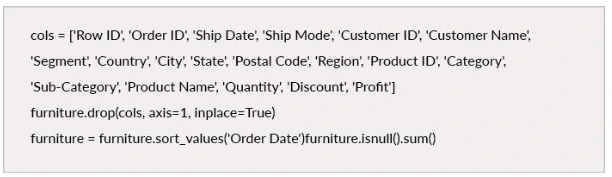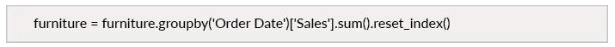### Indexing with Time Series Data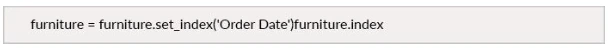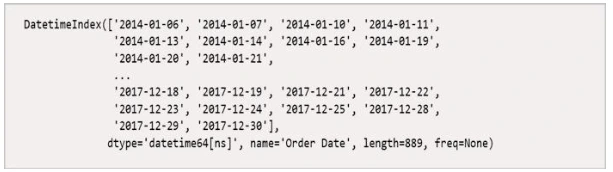The current date and time data might be tricky; hence, it is better to work with the average daily sales value for the month.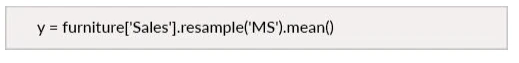Furniture sales data of 2017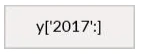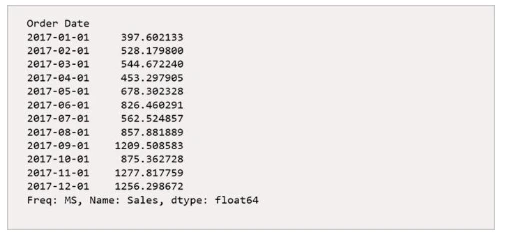### Furniture Sales Time Series Data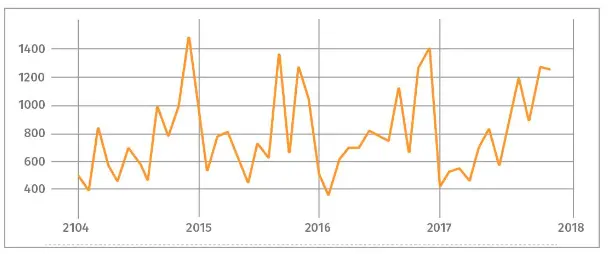# Time Series Analysis and Forecasting

Time series analysis is recording data at regular intervals. The analysis helps in forecasting future values based on past trends, which often leads to an informed decision, crucial for business.

### Case Study

An international manufacturing company was facing unpredictable volatility in the price of the primary raw material input required to manufacture its product. The client wanted to create a forecasting model to predict the price of the raw material for the next 12 months. An autoregressive time series model was developed to predict the future price, and they utilized the forecasted raw material price to monitor the cost of production, thereby increasing the profit. The model forecasted the global price of the raw material, which helped them make a better decision.

# Hire Research Optimus for Time Series Analysis and Forecasting for your Business

Research Optimus provides time series analysis and other statistical solutions to businesses of all sizes. Our data science experts analyze every dataset with accuracy and precision to help you in better decision making for your business.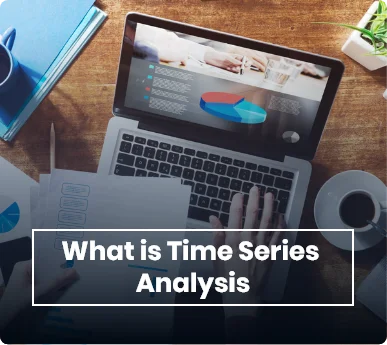Mutual Fund Managers